Courses

# Test: Ratio And Proportion- 2

## 10 Questions MCQ Test Quantitative Aptitude for GMAT | Test: Ratio And Proportion- 2

Description
This mock test of Test: Ratio And Proportion- 2 for GMAT helps you for every GMAT entrance exam. This contains 10 Multiple Choice Questions for GMAT Test: Ratio And Proportion- 2 (mcq) to study with solutions a complete question bank. The solved questions answers in this Test: Ratio And Proportion- 2 quiz give you a good mix of easy questions and tough questions. GMAT students definitely take this Test: Ratio And Proportion- 2 exercise for a better result in the exam. You can find other Test: Ratio And Proportion- 2 extra questions, long questions & short questions for GMAT on EduRev as well by searching above.
QUESTION: 1

### Gerald and James each had a few one dollar bills. After James gave 16 one dollar bills to Gerald, the ratio of the number of bills that James and Gerald respectively had was 14/11  Gerald now returned 8 one dollar bills to James, thereby increasing the ratio of the number of bills that James and Gerald respectively had to 16/9  What was the ratio of the number of bills that James and Gerald respectively had originally?

Solution:

Given:

• Let Gerald originally have g bills and James have j bills
• Number of one dollar bills that Gerald had after James gave him 16 one dollar bills = g + 16
• Number of one dollar bills that James after he gave Gerald 16 one dollar bills = j - 16
• So, we can write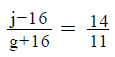• Number of one dollar bills that Gerald had after he returned 8 one dollar bills to James = g + 16 – 8
• Number of one dollar bills that James had after he got 8 one dollar bills from Gerald = j – 16 + 8
• So, we can write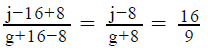To Find: J/g = ?

Approach:

1. We’re given 2 relations for j and g. Using these, we’ll:
• Either find the values of j and g, and thus solve for the required ratio.
• Or directly solve for the ratio J/g

Working out: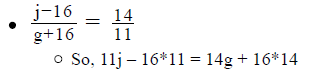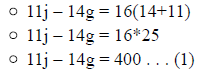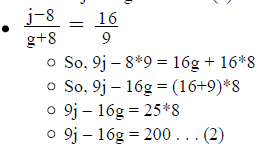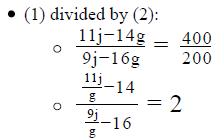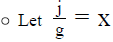• So, the above equation simplifies to: 11x-14 = 2(9x-16)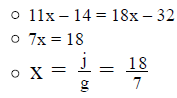Looking at the answer choices, we see that the correct answer is Option A

QUESTION: 2

### The revenue of a company is directly proportional to the number of customers it serves, which in turn is directly proportional to the marketing spend of the company. What is the revenue of the company if it spends one million dollars in marketing? (1) If the company spends \$250,000 in marketing, it earns a revenue of ten million dollars (2) The ratio of revenue of the company to the number of customers it serves is 4:1

Solution:

Step 1 & 2: Understand Question and Draw Inference

Given: Let revenue = R, Number of customers = C and marketing spend = M

• R = kC, where k is a proportionality constant
• C = pM, where p is a proportionality constant
• So, R = (kp)M . . . (I)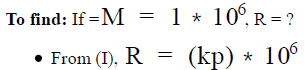• So, to answer the question, we need the value of the product of k and p

Step 3 : Analyze Statement 1 independent

1. If M = 250,000, then R = 106
• From (I), 106 = (kp)(250,000)
• From this equation, we can get the value of kp

Thus, Statement 1 alone is sufficient to answer the question.

Step 4 : Analyze Statement 2 independent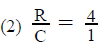• So, R = 4C
• As R = kC, we have, k = 4

Since we don’t know the value of p yet, we cannot find the value of product kp Thus, Statement 2 alone is not sufficient to answer the question.

Step 5: Analyze Both Statements Together (if needed)

Since we’ve already arrived at a unique answer in Step 3, this step is not required

QUESTION: 3

### A store stocks shirts, trousers and suits in the ratio of 4:5:6. If the store stocks only these 3 items, what is the number of items stocked in the store? (1) The number of suits is 100 more than the number of shirts stocked in the store (2) The number of suits is 150 less than the number of shirts and trousers stocked in the store

Solution:

Step 1 & 2: Understand Question and Draw Inference

Let the number of shirts, trousers and suits be 4x, 5x and 6x respectively.
Total number of items = 4x +5x+6x = 15x.

To find: 15x = ?
To answer this question, we need to find the value of x

Step 3 : Analyze Statement 1 independent

1. The number of suits is 100 more than the number of shirts stocked in the store

6x – 4x = 100
i.e. 2x = 100
x = 50

Step 4 : Analyze Statement 2 independent

2. The number of suits is 150 less than the number of shirts and trousers stocked in the store
(4x +5x) – 6x = 150
3x = 150
x = 50

Step 5: Analyze Both Statements Together (if needed)

Since, we have a unique answer from steps 3 and 4, this step is not required.

QUESTION: 4

A school library consists of literature books and science books in the ratio of 5:6. If 50 more literature books are added to the library and the number of science books is increased by 1/4th of the existing science books, the ratio of the literature books and science books increases to 4:3. If the library consists of only literature and the science books, what is the initial number of books in the library?

Solution:

Given:

• Let the number of literature books be l and the number of science books be s
• l : s = 5 : 6
• Number of literature books added = 50
• Total literature books now = l + 50
• Number of science books increased = ¼ * s
• Total science books now = s + ¼ * s
• New Ratio of literature and science books = 4 : 3

To Find: Initial number of books in the library

• Value of l + s?

Approach:

1. To Find the value of l + s, we need to find the values of l and s.
2. We know that l : s = 5 : 6. So, we need to find another relation between l and s to find unique values of l and s.
3. We are given that the new number of literature books = l + 50 and the new number of science books is s + ¼ * s. The ratio of these literature and science books is 4 : 3
• So, we have here another relation between l and s

Working out: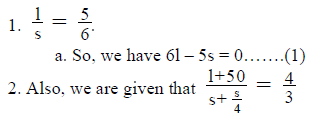a. So, we have 3l + 150 = 5s, i.e. 5s – 3l = 150…….(2)
3. Adding equations (1) and (2), we have 3l = 150, i.e. l = 50
a. Putting the value of l in equation (2), we have 5s = 150 + 150, i.e. s = 60
4. Hence, initial books in the library = l + s = 50 + 60 = 110
Thus, the library initially had 110 books in total.

QUESTION: 5

Five friends Alastair, Bell, Cook, Darren and Eoin appeared in an aptitude test. Alastair scored exactly 1/2  of Darren’s score, whose
score was 1/5th  more than Cook’s score. Eoin scored more than Cook and Darren’s score was 3/2 times that of Bell’s score. If the average score (arithmetic mean) of the group was 50, what was the range of the scores of the group?

Solution:

Given:

• Relation of scores of Alastair, Bell, Cook, Darren and Eoin in an Aptitude test
• Alastair’s score = ½ * Darren’s Score
• Darren’s Score = Cook’s score + 1/5* Cook’s score =3/2 * Bell’s score
• Eoin’s score = Cook’s score + 2/5* Cook’s score
• Average score of the group = 50
• So, total score of the group = 50*5 = 250

To Find: Range of the scores of the group

• Range of the score = Highest score – Lowest Score

Approach:

1. Range of the score = Highest score – Lowest Score
• For finding the highest and the lowest score, we need to find the individual scores of all the 5 people
2. Let’s assume the score of any one person to be x. Let’s assume that person to be Alastair
• As we are given the relation between the scores of all the 5 people, we will express the scores of other people in terms of x.
3. Once we get the scores of all the people in terms of x, we know that the sum of their scores is 250.
• So, equating the sum of the scores of all the 5 people to 250 will give us an equation in x, which will give us a unique value of x.

Working out:

1. Let Alastair’s score be x……….(1)
2. Darren’s Score
• We know that, Alastair’s score = ½ * Darren’s Score
• So, Darren’s score = 2x………..(2)
3. Cook’s Score
• Now, we know that Darren’s Score = Cook’s score + 1/5 * Cook’s score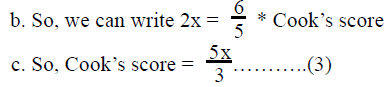4. Bell’s Score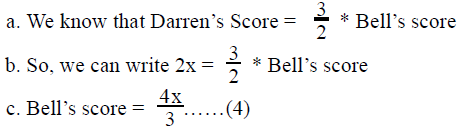5. Eoin’s Score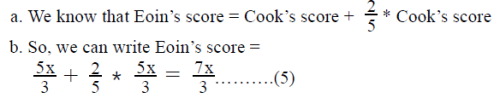6. Also, sum of all the scores = 250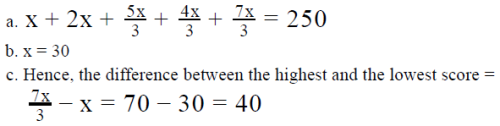So, the range of the scores of the group is 40.

QUESTION: 6

In triangle ABC, ∠ABC is 7/20  times less than ∠ACB, and ∠ACB is  2/3 times greater than ∠BAC. What is the measure of ∠BAC?

Solution:

Given:

• In ΔABC, let the measure of ∠BAC be ao , ∠ABC be bo and ∠ACB be co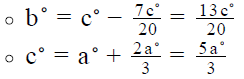To Find:

ao = ?

Approach:

1. To find the value of ao we need to draft an equation in terms of ao only. We are given the value of co in terms of ao , and using the given information, we can infer the value of bo in terms of ao .
2. We know another relation between ao, bo, co, - The Angle Sum Property. By using the values of bo and co in terms of a in the Angle Sum relation, we can find the value of ao

Working out: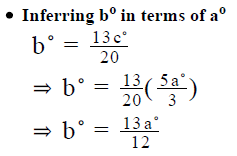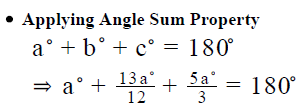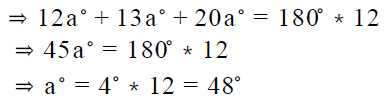Looking at the answer choices, we see that the correct answer is Option B

QUESTION: 7

The students in a class were asked to vote for their favourite subject. W students voted for mathematics, X students voted for science, Y for history and Z for literature. The ratio of W to Y was 4:5, Z to W was 5:6 and X to Z was 13:10. If each student in the class voted for one of the four subjects above, what percent of the students in the class voted for mathematics?

Solution:

Given:

• Total students in the class = W + X + Y + Z
• Favorite subject votes were as under:
• Mathematics = W
• Science = X
• History = Y
• Literature = Z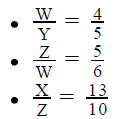To Find: % of students who voted for Mathematics as their favorite subject

Approach:

1.  % of students who voted for Mathematics as their favorite subject=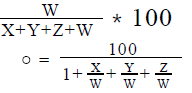2. So, to find the required percentage, we need to find the values of

​​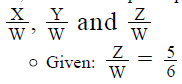• We’ll use the given ratios to find the other 2 required values

Working out:

• Finding Y/W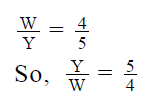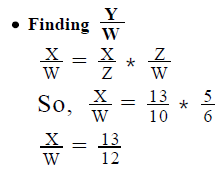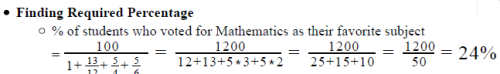Looking at the answer choices, we see that the correct answer is Option C

QUESTION: 8

2/5more water by weight is added to a salt-sugar-water solution. Before the addition of the water, what is the ratio of the weight of the water to the weight of the solution?

1. Due to the addition of water, the ratio of the weight of sugar in the solution to the weight of the solution decreases from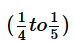.
2. After the addition of water, the ratio of the weight of water in the solution to the weight of the solution becomes 7/10
Solution:

Step 1 & 2: Understand Question and Draw Inference

Given:

Initial Solution

• Let the weight of
• salt = t
• sugar = g
• water = w
• So, weight of whole solution = w + g + t

Final Solution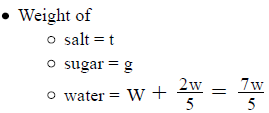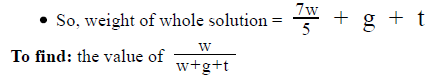Step 3 : Analyze Statement 1 independent

(1) Due to the addition of water, the ratio of the weight of sugar in the solution to the weight of the solution decreases from toInitial Solution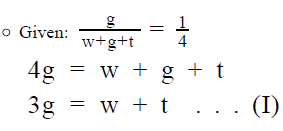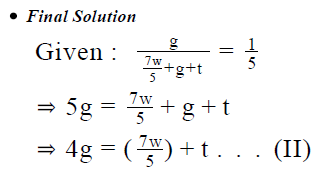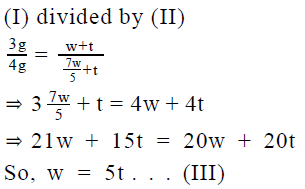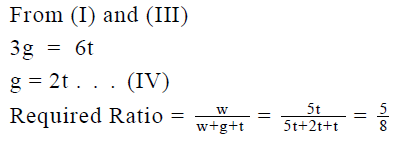Thus, Statement 1 is sufficient.

Step 4 : Analyze Statement 2 independent

(2) After the addition of water, the ratio of the weight of water in the solution to the weight of the solution becomes 7/10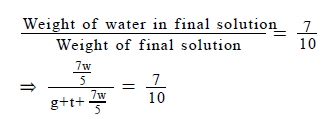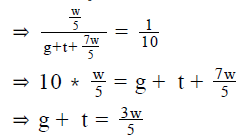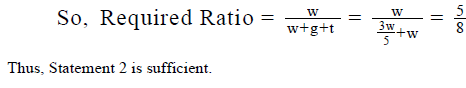Step 5: Analyze Both Statements Together (if needed)

Since we’ve already arrived at a unique answer in each of Steps 3 and 4, this step is not required

QUESTION: 9

A teacher took a pre-announced test in a class 3/4th. of the students had not prepared for the test. Of the students who had prepared for the test, 2/3rd passed the test. If only 25 percent of the class passed the test, what fraction of the students who had not prepared for the test, failed the test?

Solution:

Given:

• Number of students who did not prepare for the test =3/4 of the total student
• Number of students who prepared for the test = 1/4 of the total student
• Number of students who prepared and passed the test = 2/3of the students who prepared for the test
• Number of students who passed the test = 25% of the total students
• Number of students who failed the test = 75% of the total students

To Find: fraction of the students who had not prepared for the test and failed the test?

Approach:

1. Required Fraction =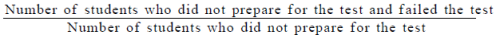• So, we need to either find the values of both “Number of students who did not prepare and failed the test” and “Number of students who did not prepare for the test” or express both of them in terms of a common variable
• As we are not given any information about the number of students, we will try to express both of them in terms of a common variable
2. Number of students who did not prepare for the test
• Let’s assume the total number of students in the class be x.
As we know that Number of students who did not prepare for the test =  3/4  of the total student, we can find the number of students who did not prepare for the test in terms of x.
• Also that would give us the number of students who prepared for the test in terms of x.
3. Number of students who did not prepare for the test and failed the test
• We know that number of students who failed the test = 75% of the total students
• Also, number of students who failed the test = Number of students who prepared and failed the test + Number of students who did not prepare and failed the test
• Now, we know that Number of students who prepared and passed the test =2/3 of the students who prepared for the test.
• So, number of students who prepared and failed the test = (Number of students who prepared) – (number of students who prepared and passed the test)
• Using the above relation, we can find the number of students who prepared and failed the test in terms of x.

Working out:

1. Number of students who did not prepare for the test = 3/4  of the total student =
• So, number of students who prepared for the test =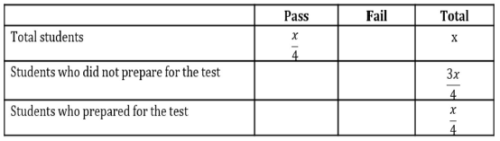2. Number of students who failed the test = 75% of x = 3/4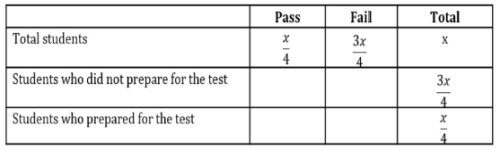3. Also, number of students who failed the test = Number of students who prepared and failed the test + Number of students who did not prepare and failed the test

• 3/4 =  Number of students who prepared and failed the test + Number of students who did not prepare and failed the test.
•  Now, we are given that Number of students who prepared and passed the test =  2/3  of the students who prepared for the test =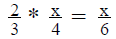• So, number of students who prepared and failed the test =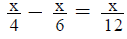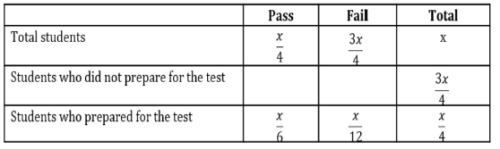• Using (3.a), (3.b) and (3.c), we can write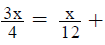Number of students who did not prepare and failed the test, which gives us the number of students who did not prepare and failed the test =  2/3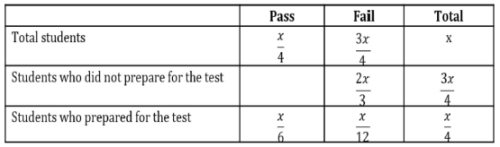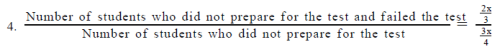QUESTION: 10

In a developing country, the price of a stock is directly proportional to the reciprocal of the inflation in the country, which is in turn directly proportional to the local diesel prices. The price of the stock is 100 currency units when the inflation is 10 units and when the inflation is 12 units, the local price of the diesel is 60 currency units. By how many currency units should the local diesel price fall so that the price of the stock, which is currently at 200 currency units, increases by 25 percent?

Solution:

Given: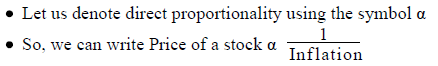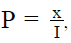where x is a constant and P and I are price of the stock and Inflation respectively

• Inflation α Local Diesel prices
• I = bD, where b is a constant and D is the local diesel price.
• P = 100 when I = 10
• I = 12 when D = 60

To Find: By how many currency units should the local diesel price fall so that the price of the stock, which is currently at 200 currency units, increases by 25 percent?

• Current Price stock = 200 currency units.
• Increased Price stock = 200 *1.25 = 250 currency units

Approach:

1. Let the current local price of diesel be D and the local price of Diesel at which the price of the stock increases by 25 percent be D’. So, the local Diesel price should fall by (D – D’) units
• So, we should find the value of D and D’ at the current price and the increased price of the stocks.
2. We need to find the relation between the price of a stock and the diesel prices
• We know the relation between price of a stock and the inflation, which is given by• We also know the relation between Inflation and local diesel prices, which is given by I = bD
3. Using the above relations, we will establish a relation between price of a stock and the local diesel prices.
4. Once, we establish a relation between price of a stock and the local diesel prices, we will need to find the values of constants b and x to calculate the change needed in local diesel prices when the price of a stock increases by 25 percent.
• As we are given the price of a stock for a particular level of inflation, we can find the value of x using the relation• Similarly, as we are given the value of inflation for a particular level of local diesel prices, we can find the value of b using the relation I = bD

Working out: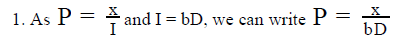2. Calculating values of x and b

• Price of a stock = 100 when inflation = 10
• Using the relationwe can write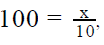which gives us x = 1000

• Inflation = 12 when the local diesel prices = 60
• Using the relation I = bD, we can write 12 =b *60, i.e. b = 1/5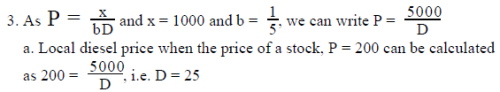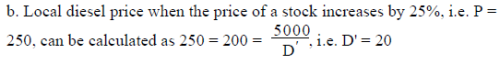4. So, the fall in local diesel price = 25 – 20 = 5
Hence, the local diesel price should fall by 5 currency units so that the price of
the stock should increase by 25% from 200.